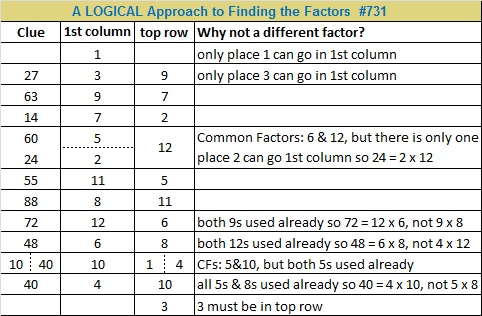# 731 and Level 6

• 731 is a composite number.
• Prime factorization: 731 = 17 x 43
• The exponents in the prime factorization are 1 and 1. Adding one to each and multiplying we get (1 + 1)(1 + 1) = 2 x 2 = 4. Therefore 731 has exactly 4 factors.
• Factors of 731: 1, 17, 43, 731
• Factor pairs: 731 = 1 x 731 or 17 x 43
• 731 has no square factors that allow its square root to be simplified. √731 ≈ 27.03711669.

Here is a Level 6 puzzle for you try:Print the puzzles or type the solution on this excel file: 12 Factors 2015-12-28

———————————————————

731 can be written as the sum of consecutive numbers 3 different ways:

• 365 + 366 = 731; that’s 2 consecutive numbers.
• 35 + 36 + 37 + 38 + 39 + 40 + 41 + 42 + 43 + 44 + 45 + 46 + 47 + 48 + 49 + 50 + 51 = 731; that’s 17 consecutive numbers.
• 5 + 6 + 7 + 8 + 9 + 10 + 11 + 12 + 13 + 14 + 15 + 16 + 17 + 18 + 19 + 20 + 21 + 22 + 23 + 24 + 25 + 26 + 27 + 28 + 29 + 30 + 31 + 32 + 33 + 34 + 35 + 36 + 37 + 38 = 731; that’s 34 consecutive numbers.

Because 17 is one of its factors,  731 is the hypotenuse of Pythagorean triple 344-645-731 making 344² + 645² = 731².

239 + 241 + 251 = 731 so 731 is the sum of 3 consecutive prime numbers.

731 is palindrome 3A3 in BASE 14; note that 3(14²) + 10(14) + 3(1) = 731.

731 is also 123 in BASE 26; note that 1(26²) + 2(26) + 3(1) = 731.

———————————————————Thanks to Ricardo whose tweet I include here: